Skip to content

Matrix Laboratory or Matlab is designed to work mainly with arrays and matrices. All the Matlab variables, irrespective of their data type, are multidirectional arrays and all matrices are two-dimensional arrays.

A matrix is a two-dimensional array that can be easily created using Matlab. There are multiple methods like magic, zeros, ones, rand, or just the direct array method for creating a matrix, depending on the type of matrix. Multiple operations can be performed on a matrix, out of which one essential operation is transposing. There are two methods to obtain the transpose of the matrix: inbuilt function ‘transpose’ or using operators.

In its true mathematical sense, a Matrix is an arrangement of several rows and columns, and it is also defined by this number. An ‘m-by-n’ or ‘m X n’ matrix means that the matrix has m-rows and n-columns, where m and n are the dimensions. Consider an example of dimensions 3×4, meaning that there are 3 rows and 4 columns in this matrix.

In this article, we’ve covered the following primary topics.

Also Read: How to make a table in MATLAB?

# How to create a matrix in MATLAB?

Since all matrices are two-dimensional arrays, to create a matrix, the user would first require knowing how an array is made in Matlab. To create a single row array containing five elements, the syntax is as follows.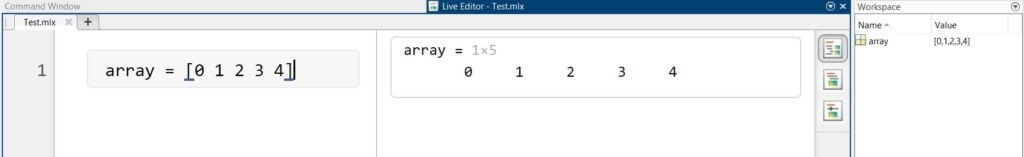Syntax (Extreme left), Output (Center), Workspace (Extreme right)

The above is considered to be a row vector, which in terms of matrices can be represented as 1×4, meaning it has one row and four columns.

## Direct method

The syntax is similar to the above, with added semicolons to separate multiple rows to create a matrix. Consider the syntax for a 3×4 matrix below.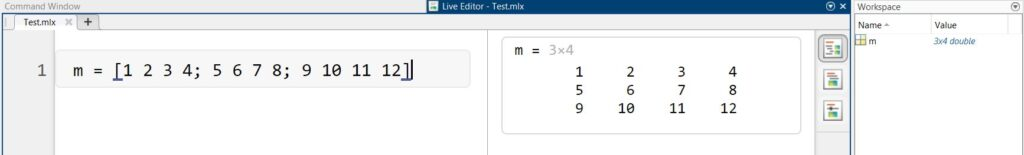Syntax (Extreme left), Output (Center), Workspace (Extreme right)

From above, it is observed that the semicolons we used as separators for separating the different rows. This method is the direct method for creating a matrix with respect to how arrays were created. This method can even be used with complex numbers like 2+5i or 9-6i and many more by replacing any of the integer values with the complex number.

## Alternative methods

The user may often require a full zero’s or one’s matrix of multiple rows and columns, or if the user has no specific set of numbers and wants random numbers for the matrix, the process could get tedious and time-taking. For such cases. Matlab has inbuilt functions to create a matrix of a specific type.

Zero’s Matrix

The syntax for a full zero matrix follows.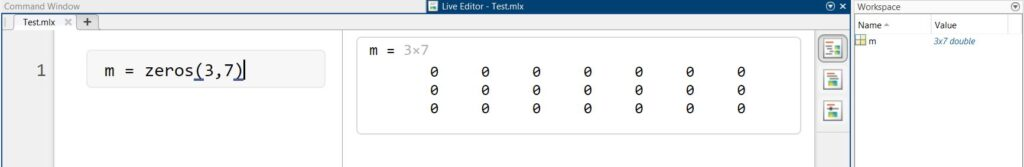Syntax (Extreme left), Output (Center), Workspace (Extreme right)

The inbuilt function used to create a zeros matrix is ‘zeros’. Note that the numbers are written in the parenthesis () represent the number of rows and columns. Here, the number of rows is 3, while columns are 7.

One’s Matrix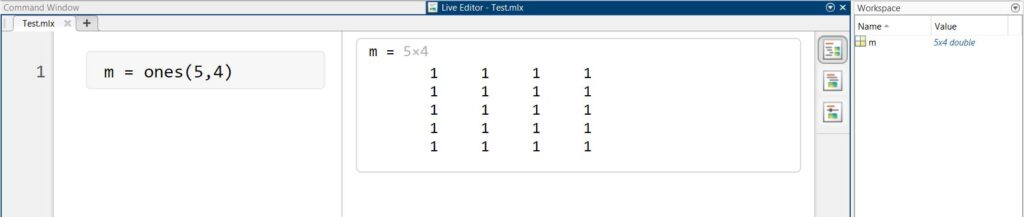Syntax (Extreme left), Output (Center), Workspace (Extreme right)

Just like the zero’s matrix, using the inbuilt function ‘ones’, a full one’s matrix is created. Here the matrix consists of 5 rows and 4 columns.

Random Matrix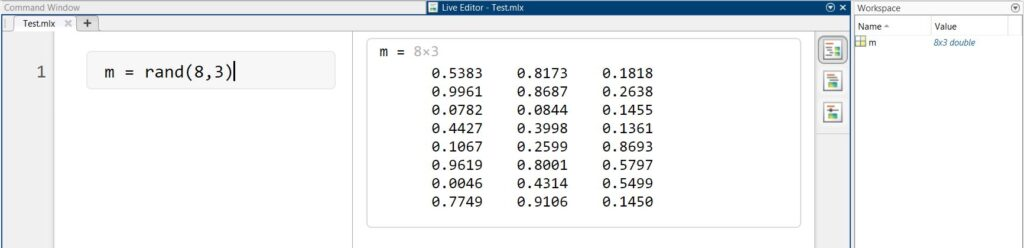Syntax (Extreme left), Output (Center), Workspace (Extreme right)

The random matrix can be created using the inbuilt function ‘rand’. This function generates a matrix of specified dimensions with random numbers. For completely experimental codes or those with no value specifications.

Random Square Matrix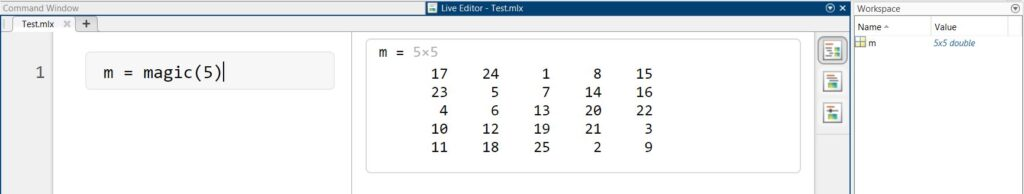Syntax (Extreme left), Output (Center), Workspace (Extreme right)

The inbuilt function ‘magic’ is used for creating a square matrix of m-by-m dimensions with randomly assigned real integer values. Note that this method cannot create a rectangular matrix — 3×4 or 7×5 and so on.

# How to transpose a matrix in MATLAB?

The transpose of a matrix is a matrix that is formed by replacing the rows with columns and vice-versa. It can also be defined as the flipping of a matrix over its diagonal. This means that if there is a 3×4 matrix, upon taking its transpose, it becomes 4×3; that is, a 3 row and 4 column matrix changes into a 4 row and 3 column matrix. If a row vector/matrix such as 1×4 is considered transpose, it becomes a column vector/matrix 4×1.

Transpose of a matrix is one of the multiple operations that can be performed on a Matlab matrix. There are two methods to transpose a matrix in Matlab.

Method 1

Here, ‘m’ is a 3×4 matrix pre-assigned. The variable ‘transm’ is assigned to the value to transpose the matrix m. The syntax specified for the transpose of a matrix is the name of the matrix — (Name-full stop-hyphen).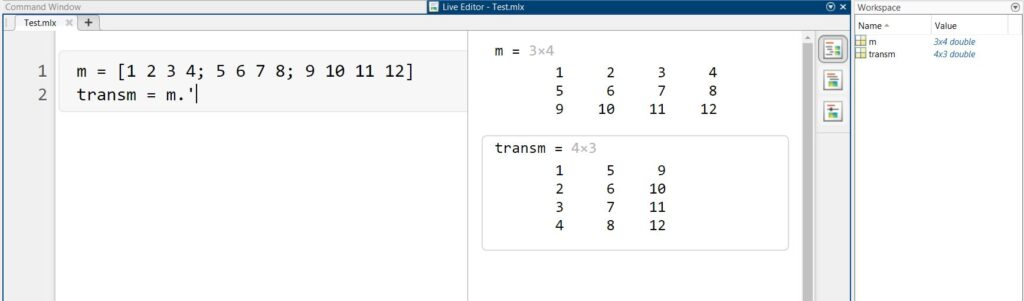Syntax (Extreme left), Output (Center), Workspace (Extreme right)

The output and workspace section observes that ‘transm’ is a 4×3 matrix while ‘m’ was a 3×4 matrix.

Method 2

Here, the inbuilt function ‘transpose’ is used to obtain ‘transm’, the transpose of the matrix ‘m’.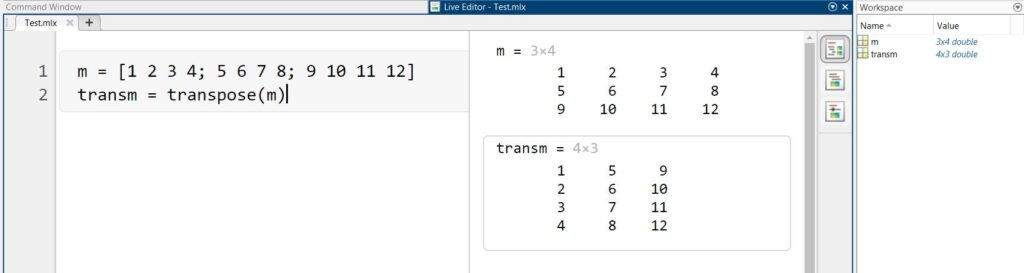Syntax (Extreme left), Output (Center), Workspace (Extreme right)

The output observed is exactly the same as that obtained in Method 1.

Both Method 1 and Method 2 can be used for a matrix containing complex numbers as well. The output will still be a perfect transpose of the matrix and there will be no change of sign in the complex number.

## Hello There!

### If you like what you read, please support our publication by sharing it with your friends, family and colleagues. If you're running an Adblocker, we humbly request you to whitelist us.

Copyright © 2018-2021 Candid.Technology | Candid Today Press Pvt. Ltd.​